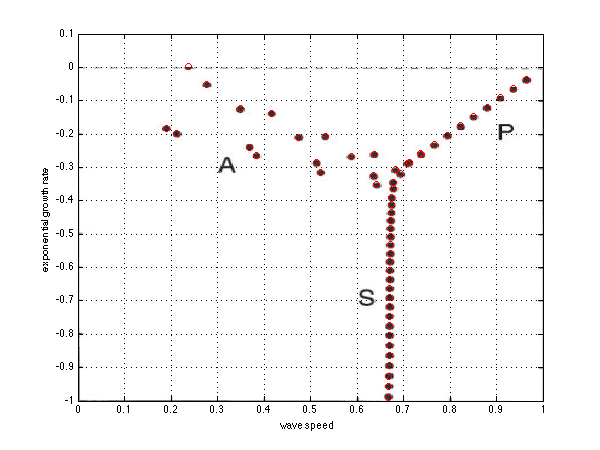# Stability of the plane Poiseuille flow in primitive variables

In this code, we build the stability matrix for the Poiseuille flow. This is the flow in a flat channel between two infinite plates, driven by a pressure gradient. Here is a sketch of the flow:Sketch of the Poiseuille flow.

We do this is the primitive variables u,v and p the horizontal and vertical components of the velocity and the pressure. Thus we use the Navier-Stokes equations, linearized about a parabolic velocity profile, plus as well the continuity equation. Since there is no time derivative in the continuity equation for incompressible flow this system becomes a descriptor system, also known as differential algebraic equation. This is a nice formulation because there are no fourth order derivative as for the Orr-Sommerfeld equation.

The parameters are the wave number \alpha=2\pi/\lambda where \lambda is the wavelength in the direction of the flow, the height of the domain L which must be 2, the Reynolds number and the number of grid points.

clear all; clf;

alpha=1;    % the wave number
L=2;        % the height of the domain, from -1 to 1
Re=10000;    % the Reynolds number
n=200;      % the number of grid points

% differentiation and integration
scale=-2/L;
[y,DM] = chebdif(n,2);
D=DM(:,:,1)*scale;
DD=DM(:,:,2)*scale^2;
y=y/scale;
Z=zeros(n,n); I=eye(n);
INT=([diff(y)',0]+[0,diff(y)'])/2;


# Differentiation matrices

We need to compute the derivatives in x and also in y. But in fact as is usually done for stability of parallel flows, we can do a Fourier transform in the direction where the system does not change, so here the numerical differentiation is done in y, and the differentiation in x simply ammounts to multiplication with i\alpha.

% renaming the differentiation matrices
I=eye(n); Z=zeros(n,n);
dy=D; dyy=DD;
dx=i*alpha*I; dxx=-alpha^2*I;
Delta=dxx+dyy;

% base flow
U=1-y.^2; Uy=-2*y;
S=-diag(U)*dx+Delta/Re;


# System’s matrix

Here we build the dynamics matrices A and E. We can see in A how the pressure comes into the equation through just its gradient. The last row of A corresponds tothe continuity equation u_x+v_y=0. To enforce that the divergence of the velocity field be zero, this equation must be zero on the left hand side, we write \displaystyle sEq=Aq where q is the eigenvector composed like this \displaystyle q=\left(\begin{array}{c} u \\ v \\ p \end{array}\right) and s is the eigenvalue. And E is like this \displaystyle E=\left(\begin{array}{ccc} I & 0 & 0 \\ 0 & I & 0 \\ 0 & 0 & 0 \\ \end{array}\right) so the last row is full of zeros! This is why E is not invertible and the system is a Differential algebraic system instead of just a dynamic systeM

% the matrices
A=[ ...
S, -diag(Uy), -dx; ...
Z, S, -dy; ...
dx, dy, Z];
E=blkdiag(I,I,Z);


# Locations on the grid

For easily imposing the boundary condition, we first define the locations on the grid of the u velocity as well as for v and p. This step is not very important here, whereas it is important with more variables, and especially usefull in 2D.

% locations on the grid
u=1:n; v=u+n; p=v+n;

# Boundary conditions

% boundary conditions
III=eye(3*n);
loc=[u(1) u(n) v(1) v(n) ];
C=III(loc,:);
E(loc,:)=0;  A(loc,:)=C;


# Computing the eigenmodes

We can use the eig function for DAE just as for dynamic systems. the difference is that we will get infinite eigenvalues. Thinking of the incompressible system as the limit of the compressible one, you realize that these infinite eigenvalues are the limit of the sound modes. We remove them, we sort the eigenvalues and eigenvectors.

% computing eigenmodes
[U,S]=eig(A,E);
s=diag(S);  [t,o]=sort(-real(s)); s=s(o); U=U(:,o);
rem=abs(s)>1000; s(rem)=[]; U(:,rem)=[];


# Figure for comparison

Here we do not have a theory for validation of our computation, so we digitize a figure from Schmid & Henningson’s book “Stability and transition in shear flows”. The Reynolds is 10000. It is nice to plot our results directly on figures from the litterature.

% loading the scanned figure for comparison and plotting
x=linspace(0,1,ss(2));
y=linspace(0,-1,ss(1));
image(x,y,a); axis xy
hold on

plot(-imag(s)/alpha,real(s),'ro')
grid on
axis([0,1,-1,0.1]);
xlabel('wave speed'); ylabel('exponential growth rate')


We can see that our results nicely fit with the book (and that the flow is unstable, since one of the eigenmodes has a positive growth rate).The spectrum of the Poiseuille flow at Reynolds 10000

# Figure for eigenmode structrure

figure(2);
eigenmode = U(:,1); % first eigenmode
plot(real(eigenmode(u)),y,'r-',imag(eigenmode(u)),y,'r--',real(eigenmode(v)),y,'b-',imag(eigenmode(v)),y,'b--');
xlabel('u,v');ylabel('y');
legend('Re(u)','Im(u)','Re(v)','Im(v)');
legend('Location','East');
grid on;

set(gcf,'paperpositionmode','auto');
print('-dpng','-r100','PlanePoiseuille_Mode_Re10000_k1.png');Structure of the most amplified mode of the Plane Poiseuille flow at Re = 10000, k=1

# Figure for the Reynolds stress

Tauxy = eigenmode(u).*conj(eigenmode(v))+eigenmode(u).*conj(eigenmode(v));
figure(3);
plot(y,Tauxy);
legend('Re(u)','Im(u)','Re(v)','Im(v)');
legend('Location','East');
xlabel('\tau_{xy},\tau_{xy} * U''');ylabel('y');
grid on;

set(gcf,'paperpositionmode','auto');
print('-dpng','-r100','PlanePoiseuille_Tauxy.png');Reynolds stress for Re = 10000, k=1

Note that the reynolds stress defined as $_{xy} = -< u’ v’>$ is of the same sign as the base flow gradient U'. There is a sign confusion in Huerre & Rossi and in Charru when reproducing the result of Stuart (1963)